## Last Chance: RW Accelerator Bootcamps

Bootcamps start August 15th — Don't miss out!
Save 50% and go from novice coder to job-ready mobile developer in just 12 weeks.

# RxSwift: Transforming Operators

Learn how to leverage transforming operators in RxSwift, in this tutorial taken from our latest book, RxSwift: Reactive Programming With Swift!

In this tutorial, you’re going to learn about one of the most important categories of operators in RxSwift: transforming operators. You’ll use transforming operators all the time, to prep data coming from an observable for use by your subscriber.

Once again, there are parallels between transforming operators in RxSwift and the Swift standard library, such as `map(_:)` and `flatMap(_:)`. By the end of this tutorial, you’ll be transforming all the things!

## Getting Started

The starter project for this tutorial is named RxSwiftPlayground; you can download it here. Once you’ve opened it and done an initial build, you’re ready for action.

## Transforming Elements

Observables emit elements individually, but you will frequently want to work with collections, such as when you’re binding an observable to a table or collection view, which you’ll learn how to do later in the book. A convenient way to transform an observable of individual elements into an array of all those elements is by using `toArray`. As depicted in this marble diagram, `toArray` will convert an observable sequence of elements into an array of those elements, and emit a `.next` event containing that array to subscribers.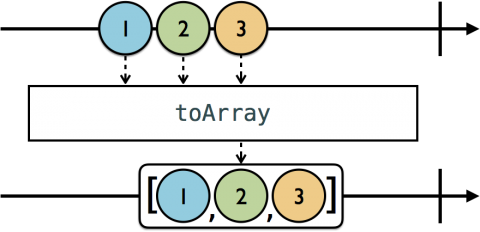Add this new example to your playground:

```example(of: "toArray") {

let disposeBag = DisposeBag()

// 1
Observable.of("A", "B", "C")
// 2
.toArray()
.subscribe(onNext: {
print(\$0)
})
}
```

Here’s what you just did:

1. Create an observable of letters.
2. Use `toArray` to transform the elements in an array.

An array of the letters is printed.

```--- Example of: toArray ---
["A", "B", "C"]
```

RxSwift’s `map` operator works just like Swift’s standard `map`, except it operates on observables. In the marble diagram, `map` takes a closure that multiplies each element by `2`.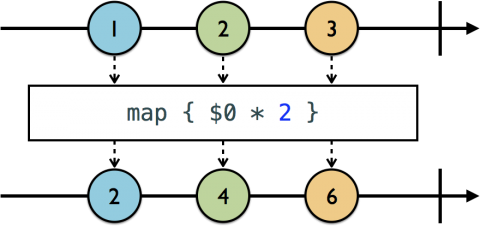```example(of: "map") {

let disposeBag = DisposeBag()

// 1
let formatter = NumberFormatter()
formatter.numberStyle = .spellOut

// 2
Observable<NSNumber>.of(123, 4, 56)
// 3
.map {
formatter.string(from: \$0) ?? ""
}
.subscribe(onNext: {
print(\$0)
})
}
```

Here’s the play-by-play:

1. You create a number formatter to spell out each number.
2. You create an observable of `NSNumber`s (so that you don’t have to convert integers when using the formatter next).
3. You use `map`, passing a closure that gets and returns the result of using the formatter to return the number’s spelled out string or an empty string if that operation returns `nil`.

Chapter 5 of the book covers filtering operators, some of them with `withIndex` variations. The same holds true for transforming operators. `mapWithIndex` also passes the element’s index to its closure. In this marble diagram, `mapWithIndex` will transform the element by multiplying it by `2` if its index is greater than `1`, otherwise it will pass through the element as-is, so only the 3rd element is transformed.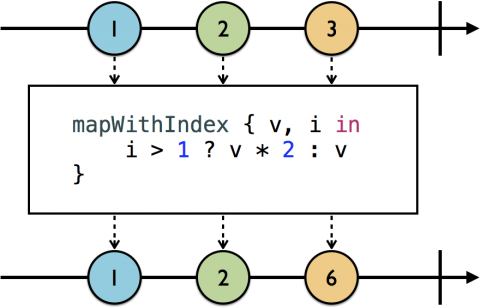Now add this new example to your playground to implement the example in the marble diagram:

```example(of: "mapWithIndex") {

let disposeBag = DisposeBag()

// 1
Observable.of(1, 2, 3, 4, 5, 6)
// 2
.mapWithIndex { integer, index in
index > 2 ? integer * 2 : integer
}
.subscribe(onNext: {
print(\$0)
})
}
```

Quite simply:

1. You create an observable of integers.
2. You use `mapWithIndex`, and if the element’s `index` is greater than `2`, multiply it by `2` and return it, else return it as is.

Only the fourth element onward will be transformed and sent to the subscriber to be printed.

```--- Example of: mapWithIndex ---
1
2
3
8
10
12
```

You may have wondered at some point, “How do I work with observables that are properties of observables?” Enter the matrix.

## Transforming Inner Observables

Add the following code to your playground, which you’ll use in the upcoming examples:

```struct Student {

var score: Variable<Int>
}
```

`Student` is structure that has a `score` property that is a `Variable`. RxSwift includes a few operators in the `flatMap` family that allow you to reach into an observable and work with its observable properties. You’re going to learn how to use the two most common ones here.

Note: A heads up before you begin: these operators have elicited more than their fair share of questions (and groans and moans) from newcomers to RxSwift. They may seem complex at first, but you are going to walk through detailed explanations of each, so by the end of section you’ll be ready to put these operators into action with confidence.

The first one you’ll learn about is `flatMap`. The documentation for `flatMap` describes that it “Projects each element of an observable sequence to an observable sequence and merges the resulting observable sequences into one observable sequence.” Whoa! That description, and the following marble diagram, may feel a bit overwhelming at first. Read through the play-by-play explanation that follows, referring back to the marble diagram.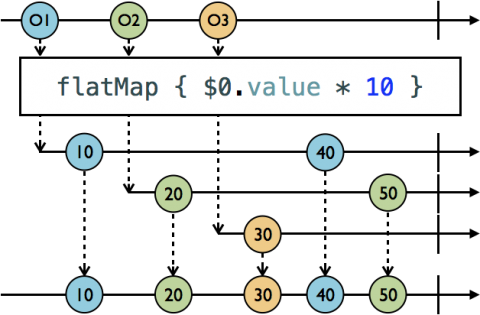The easiest way to follow what’s happening in this marble diagram is to take each path from the source observable (the top line) all the way through to the target observable that will deliver elements to the subscriber (the bottom line). The source observable is of an object type that has a `value` property that itself is an observable of type `Int`. It’s `value` property’s initial value is the number of the object, that is, `O1`’s initial `value` is `1`, `O2`’s is `2`, and `O3`’s is `3`.

Starting with `O1`, `flatMap` receives the object and reaches in to access its `value` property and multiply it by `10`. It then projects the transformed elements from `O1` onto a new observable (the 1st line below `flatMap` just for `O1`), and that observable is flattened down to the target observable that will deliver elements to the subscriber (the bottom line).

Later, `O1`’s `value` property changes to `4`, which is not visually represented in the marble diagram (otherwise the diagram would become even more congested). But the evidence that `O1`’s `value` has changed is that it is transformed, projected onto the existing observable for `O1` as `40`, and then flattened down to the target observable. This all happens in a time-linear fashion.

The next value in the source observable, `O2`, is received by `flatMap`, its initial value `2` is transformed to `20`, projected onto a new observable for `O2`, and then flattened down to the target observable. Later, `O2`’s `value` is changed to `5`. It is transformed to `50`, projected, and flattened to the target observable.

Finally, `O3` is received by `flatMap`, its initial `value` of `3` is transformed, projected, and flattened.

`flatMap` projects and transforms an observable value of an observable, and then flattens it down to a target observable. Time to go hands-on with `flatMap` and really see how to use it. Add this example to your playground:

```example(of: "flatMap") {

let disposeBag = DisposeBag()

// 1
let ryan = Student(score: Variable(80))
let charlotte = Student(score: Variable(90))

// 2
let student = PublishSubject<Student>()

// 3
student.asObservable()
.flatMap {
\$0.score.asObservable()
}
// 4
.subscribe(onNext: {
print(\$0)
})
}
```

Here’s the play-by-play:

1. You create two instances of `Student`, `ryan` and `charlotte`.
2. You create a source subject of type `Student`.
3. You use `flatMap` to reach into the `student` subject and access its `score`, which is a `Variable`, so you call `asObservable()` on it. You don’t modify `score` in any way. Just pass it through.
4. You print out `.next` event elements in the subscription.

Nothing is printed yet. Add this code to the example:

```student.onNext(ryan)
```

As a result, `ryan`’s `score` is printed out.

```--- Example of: flatMap ---
80
```

Now change `ryan`’s `score` by adding this code to the example:

```ryan.score.value = 85
```

`ryan`’s new `score` is printed.

```85
```

Next, add a different `Student` instance (`charlotte`) onto the source subject by adding this code:

```student.onNext(charlotte)
```

`flatMap` does its thing and `charlotte`’s `score` is printed.

```90
```

Here’s where it gets interesting. Change `ryan`’s score by adding this line of code:

```ryan.score.value = 95
```

`ryan`’s new `score` is printed.

```95
```

This is because `flatMap` keeps up with each and every observable it creates, one for each element added onto the source observable. Now change `charlotte`’s `score` by adding the following code, just to verify that both observables are being monitored and changes projected:

```charlotte.score.value = 100
```

Sure enough, her new `score` is printed out.

```100
```

To recap, `flatMap` keeps projecting changes from each observable. There will be times when you want this behavior. And there will be times when you only want to keep up with the latest element in the source observable. So what do you think is the name of the `flatMap` operator that only keeps up with the latest element?

### flatMapLatest

`flatMapLatest` is actually a combination of two operators, `map` and `switchLatest`. You’ll learn about `switchLatest` in the “Combining Operators” chapter of the book, but you’re getting a sneak peek here. `switchLatest` will produce values from the most recent observable, and unsubscribe from the previous observable.

So, `flatMapLatest` “Projects each element of an observable sequence into a new sequence of observable sequences and then transforms an observable sequence of observable sequences into an observable sequence producing values only from the most recent observable sequence.” Wowza! Take a look at the marble diagram of `flatMapLatest`.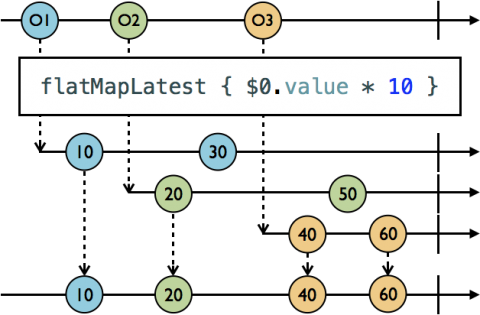`flatMapLatest` works just like `flatMap` to reach into an observable element to access its observable property, it applies a transform and projects the transformed value onto a new sequence for each element of the source observable. Those elements are flattened down into a target observable that will provide elements to the subscriber. What makes `flatMapLatest` different is that it will automatically switch to the latest observable and unsubscribe from the the previous one.

In the above marble diagram, `O1` is received by `flatMapLatest`, it transforms its `value` to `10`, projects it onto a new observable for `O1`, and flattens it down to the target observable. Just like before. But then `flatMapLatest` receives `O2` and it does its thing, switching to `O2`’s observable because it’s now the latest.

When `O1`’s `value` changes, `flatMapLatest` actually still does the transform (something to be mindful of if your transform is an expensive operation), but then it ignores the result. The process repeats when `O3` is received by `flatMapLatest`. It then switches to its sequence and ignores the previous one (`O2`). The result is that the target observable only receives elements from the latest observable.

Add the following example to your playground, which is a copy/paste of the previous example except for changing `flatMap` to `flatMapLatest`:

```example(of: "flatMapLatest") {

let disposeBag = DisposeBag()

let ryan = Student(score: Variable(80))
let charlotte = Student(score: Variable(90))

let student = PublishSubject<Student>()

student.asObservable()
.flatMapLatest {
\$0.score.asObservable()
}
.subscribe(onNext: {
print(\$0)
})

student.onNext(ryan)

ryan.score.value = 85

student.onNext(charlotte)

// 1
ryan.score.value = 95

charlotte.score.value = 100
}
```

Only one thing to point out here that’s different from the previous example of `flatMap`:

1. Changing `ryan`’s score here will have no effect. It will not be printed out. This is because `flatMapLatest` has already switched to the latest observable, for `charlotte`.
```--- Example of: flatMapLatest ---
80
85
90
100
```

So you may be wondering when would you use `flatMap` for `flatMapLatest`? Probably the most common use case is using `flatMapLatest` with networking operations. You will go through examples of this later in the book, but for a simple example, imagine that you’re implementing a type-ahead search. As the user types each letter, `s`, `w`, `i`, `f`, `t`, you’ll want to execute a new search and ignore results from the previous one. `flatMapLatest` is how you do that.

## Challenges

Completing challenges helps drive home what you learned in this tutorial. There are starter and finished versions of the challenge in the exercise files download.

### Challenge 1: Accept Alpha-Numeric Characters

In the accompanying challenge, you have code necessary to look up a contact based on a 10-digit number entered by the user.

```input
.skipWhile { \$0 == 0 }
.filter { \$0 < 10 }
.take(10)
.toArray()
.subscribe(onNext: {
let phone = phoneNumber(from: \$0)
if let contact = contacts[phone] {
print("Dialing \(contact) (\(phone))...")
} else {
}
})
```

Your goal for this challenge is to modify this implementation to be able to take letters as well, and convert them to their corresponding number based on a standard phone keypad (`abc` is 2, `def` is `3`, and so on).

The starter project includes a helper closure to do the conversion:

```let convert: (String) -> UInt? = { value in
if let number = UInt(value),
number < 10 {
return number
}

let convert: [String: UInt] = [
"abc": 2, "def": 3, "ghi": 4,
"jkl": 5, "mno": 6, "pqrs": 7,
"tuv": 8, "wxyz": 9
]

var converted: UInt? = nil

convert.keys.forEach {
if \$0.contains(value.lowercased()) {
converted = convert[\$0]
}
}

return converted
}
```

And there are closures to format and “dial” the contact if found (really, just print it out):

```let format: ([UInt]) -> String = {
var phone = \$0.map(String.init).joined()

phone.insert("-", at: phone.index(
phone.startIndex,
offsetBy: 3)
)

phone.insert("-", at: phone.index(
phone.startIndex,
offsetBy: 7)
)

return phone
}

let dial: (String) -> String = {
if let contact = contacts[\$0] {
return "Dialing \(contact) (\(\$0))..."
} else {
}
}
```

These closures allow you to move the logic out of the subscription, where it really doesn’t belong. So what’s left to do then? You’ll use multiple `map`s to perform each transformation along the way. You’ll use `skipWhile` to skip `0`s at the beginning.

You’ll also need to handle the optionals returned from `convert`. To do so, you can use a handy operator from the RxSwiftExt repo created by fellow author Marin: `unwrap`. RxSwiftExt includes useful operators that are not part of the core RxSwift library. The `unwrap` operator replaces the need to do this:

```Observable.of(1, 2, nil, 3)
.flatMap { \$0 == nil ? Observable.empty() : Observable.just(\$0!) }
.subscribe(onNext: {
print(\$0)
})
```

With `unwrap`, you can just do this:

```Observable.of(1, 2, nil, 3)
.unwrap()
.subscribe(onNext: {
print(\$0)
})
```

The starter project also includes code to test your solution. Just add your solution right below the comment `// Add your code here`.

## Where to Go From Here?

If you enjoyed what you learned in this tutorial, why not check out the complete RxSwift book, available on our store?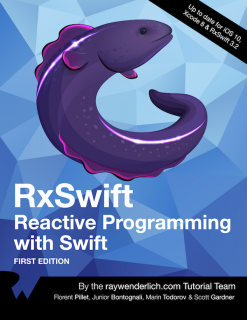Here’s a taste of what’s in the book:

• Getting Started: Get an introduction to the reactive programming paradigm, learn the terminology involved and see how to begin using RxSwift in your projects.
• Event Management: Learn how to handle asynchronous event sequences via two key concepts in Rx — Observables and Observers.
• Being Selective: See how to work with various events using concepts such as filtering, transforming, combining, and time operators.
• UI Development: RxSwift makes it easy to work with the UI of your apps using RxCocoa, which provides an integration of both UIKit and Cocoa.
• Intermediate Topics: Level up your RxSwift knowledge with chapters on reactive networking, multi-threading, and error handling.